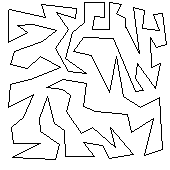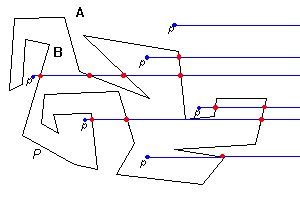# The Jordan Curve Theorem for Polygons

by Octavian Cismasu

presented to Prof. Godfried T. Toussaint
308-507A - Computational Geometry -- Web Project
Fall 1997
McGill University

### Introduction

When we ask someone to think of a polygon and describe it, in most cases we get answers like "a hexagon" or "a square" or, more generally, a convex figure and in few cases, a basic concave quadrilateral. Let's move away from these simple examples of polygons for a while and start thinking of non intersecting closed curves, as intricate or as simple as they may be. Moreover, let's think of many such curves in the plane, or simple closed curves as they are named. What property do all these curves have in common? Take one of these curves and call it C. An apparently straightforward property is that all curves similar to C represent boundaries for two sets of points, let's call them A and B, the inside and outside set respectively. Also, let A denote the set of points inside C and B the set of points outside C. Another property can be derived which is that if we join any point in A with any point in B, we have to cross the boundary between these sets, which is C.Fig. 1 - Which points of the plane are inside the polygon?
Moreover, points in the same set can always be joined by a curve lying entirely in that set. These properties seem, as I said above, very straightforward, again, when thinking about a square or a hexagon or generally, about a convex simple closed curve, but looking at a more intricate figure of a simple polygon for example, things can get confusing. For example, in Fig. 1, which is the inside and which is the outside of the represented polygon?

The human eye, after staring for a few seconds at a picture like in Fig. 1, can distinguish the two above mentioned sets A and B. One question that raises is, can a computer determine the two sets A and B in a reasonable amount of time and using a reasonable amount of resources. The answer is yes. Algorithms for point location have been developed and they are quite efficient. The object of this page is to demonstrate a theorem encapsulating some of the properties mentioned above and to show an implementation of a point-in-polygon-testing algorithm, namely, the plumb-line algorithm.

### About The Jordan Curve Theorem

##### The Theorem
Any simple closed curve C divides the points of the plane not on C into two distinct domains (with no points in common) of which C is the common boundary.
We shall take the case where C is a closed polygon P.

##### Some History
The theorem was first stated by Camille Jordan (1838 -1922) in his Cours d'Analyse. Proving this theorem has not been an easy task. The proof given by Jordan himself was quite complicated and it turned out to be invalid. The difficulty in proving this theorem lies in the generality of the concept of "simple closed curve", which is not restricted to the class of polygons or "smooth" curves, but includes all curves which are topological images of a circle. Also, concepts like inside and outside, even though they are intuitively clear, must be defined precisely before a rigorous proof is possible.

Proving
the Theorem
As important as it is to thoroughly analyze these concepts before attempting to prove this theorem, we should also remember to keep this analysis as simple as possible in order to avoid some difficulties that could arise from a too general view of our working domain. To give a concrete example, it happens that this theorem has a simple proof for the case of polygons, which are a class of simple curves that occurs very often in most important problems. Therefore, proving the theorem for this particular case (a much simpler task than proving it for the case of general simple closed curves) may be of great use in solving a lot of other important problems.

### Two Different Approaches for Proving this Theorem

The Plumbline This approach suggests the following idea: choose any fixed direction in the plane. Lead a half-line in that direction, starting at any point p in the same plane with our polygon P. Then this ray will intersect the edges of the polygon an even number of times if p is outside the polygon or an odd number of times if p is inside
Order of a Point The order of a point q with respect to a polygon P (not passing through q) is defined as the number of revolutions made by an arrow joining q to a point p on P, as p is moved once around along the boundary of P. Then if q has an even order with respect to P, it is outside and if its order is an odd number, it is inside P

### Proof of the Jordan Curve Theorem

Note: we borrow the terminology and explain the proof that appeared in "What is Mathematics".

Consider a simple polygon P. We will show that the points of the plane are divided into two sets A and B, having the following property: any two points belonging to the same set can be joined by a polygonal chain which does not cross P; also, any two points belonging to different sets cannot be joined by a polygonal chain such that this chain does not intersect P. Note that the common boundary of A and B is our polygon P. In this case, we will let the set A correspond to the outside set and the set B to the inside set.

Proof steps:

1. Chose a fixed direction in the plane; define the parity of a point.
2. Prove that if any point p1 of A is joined to any point p2 of B by a polygonal path, then this path must intersect P.
3. Prove that any two points of the same set (A or B) can be joined by a polygonal path not intersecting P.

The proof begins with chosing a fixed direction in the plane. Let this direction be non-parallel to any of the edges of P. This can always be done since P has a finite number of edges.The sets A and B can now be defined in the following way. Consider a point p and the ray through it, in the fixed direction we chose at the previous step. Then the point p belongs to the set A, if this ray intersects P an even number of times. On the other hand, p belongs to B if the ray intersects our polygon P an odd number of times.

We define the concept of parity in the following way: two points have the same parity if they belong to the same set, either A or B
We now make the following note: all points on a segment not intersecting P, have the same parity. This is obvious:

• chose a fixed direction in the plane
• consider a ray in this fixed direction
• a point p moving along this ray will have its parity changed only when the ray intersects our polygon at one of its vertices v. But even here, the parity of the point won't actually change, because of the following rule that we make up:  we will count an intersection only when the two edges of P that come together at v, are on different sides of the ray. We will not consider as an intersection the case where the two edges of P meeting at v are on the same side of the ray.

This implies that if any point p1 of A is joined to any point p2 of B by a polygonal path, then this path must intersect P because otherwise, all the points on this path (and p1 and p2 in particular) would have the same parity
Now we direct our attention to the last step in our proof, where we show that any two points of the same category (A or B) can be joined by a path which doesn't intersect P

• consider any two points p and q belonging to the same set, A or B.
• if the line segment from p to q does not intersect P, we have the desired path and we're done.
• else, consider the first intersection of this segment with P. Call this point p'. Also, consider the last intersection ofthe segment with P and call that point q'. We now construct a path starting at p and along the segment pq. Before we reach p', our path separates from pq and from the boundary of P but continues along this boundary until it reaches the continuation of pq. Finally, it comes back to q'. Does this path actually intersect pq between q' and q? If we can show that this is the case, then we have the desired path from p to q, which does not intersect P. This path will be obtained by continuing the original path along q'q until q is reached. Let's stop for a moment and note that any two points r and s close enough, but on opposite sides of an edge of P, always have different parity. This is true since the ray from one point (say r) will intersect P in one more point than the ray from the other point (say q). Back to our case, the parity changes as our path crosses pq between q' and q, since p, q and all other points on this path have the same parity. Therefore we have an intersection point between q' and q, so we can obtain a path between p and q, which does not intersect P. Since p and q belong to the same set (from our original supposition), the theorem for the case of polygons is now proved.

We can now conclude that A is the outside region of P and B, the inside of P. It follows that for any simple polygon, no matter its shape, we can say if a point is inside or outside the poylgon. Just draw a ray from this point and count the intersections with the polygon. An odd number of intersections means our tested point was inside and an even number of intersection means the point was outside the polygon. See it happening in this Java applet

### Related Material

References
1. R. Courant and H. Robbins - What is Mathematics?

2. Oxford University Press, 1941.

3. J.O'Rourke - Computational Geometry in C

4. Cambridge University Press, 1994.

Computational